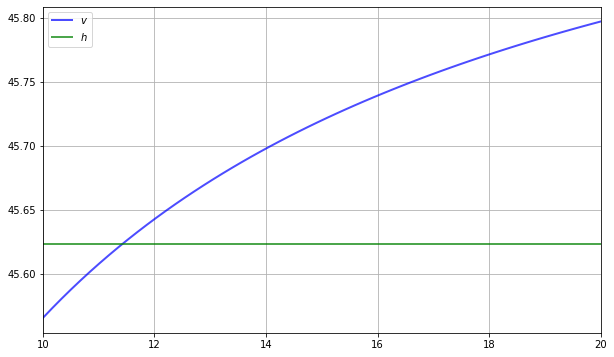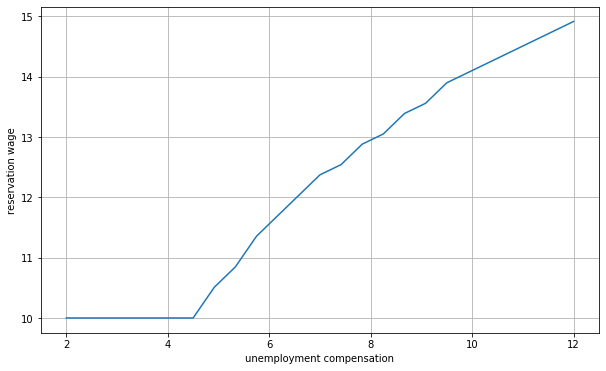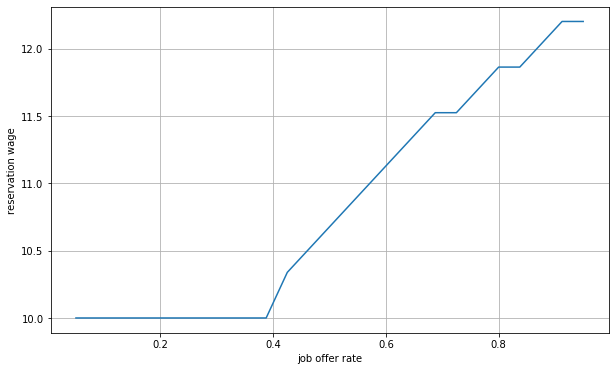How to read this lecture...

Code should execute sequentially if run in a Jupyter notebook

# Job Search II: Search and Separation¶

## Contents¶

In addition to what’s in Anaconda, this lecture will need the following libraries:

In :
!pip install --upgrade quantecon


## Overview¶

Previously we looked at the McCall job search model [McC70] as a way of understanding unemployment and worker decisions.

One unrealistic feature of the model is that every job is permanent.

In this lecture, we extend the McCall model by introducing job separation.

Once separation enters the picture, the agent comes to view

• the loss of a job as a capital loss, and
• a spell of unemployment as an investment in searching for an acceptable job

We’ll need the following imports

In :
import numpy as np
from quantecon.distributions import BetaBinomial
from numba import njit
import matplotlib.pyplot as plt
%matplotlib inline


## The Model¶

The model concerns the life of an infinitely lived worker and

• the opportunities he or she (let’s say he to save one character) has to work at different wages
• exogenous events that destroy his current job
• his decision making process while unemployed

The worker can be in one of two states: employed or unemployed.

He wants to maximize

$${\mathbb E} \sum_{t=0}^\infty \beta^t u(Y_t) \tag{1}$$

The only difference from the baseline model is that we’ve added some flexibility over preferences by introducing a utility function $u$.

It satisfies $u'> 0$ and $u'' < 0$.

### Timing and Decisions¶

Here’s what happens at the start of a given period in our model with search and separation.

If currently employed, the worker consumes his wage $w$, receiving utility $u(w)$.

If currently unemployed, he

• receives and consumes unemployment compensation $c$
• receives an offer to start work next period at a wage $w'$ drawn from a known distribution $\phi$

He can either accept or reject the offer.

If he accepts the offer, he enters next period employed with wage $w'$.

If he rejects the offer, he enters next period unemployed.

When employed, the agent faces a constant probability $\alpha$ of becoming unemployed at the end of the period.

(Note: we do not allow for job search while employed—this topic is taken up in a later lecture)

## Solving the Model using Dynamic Programming¶

Let

• $v(w)$ be the total lifetime value accruing to a worker who enters the current period employed with wage $w$
• $h$ be the total lifetime value accruing to a worker who is unemployed this period

Here value means the value of the objective function (1) when the worker makes optimal decisions at all future points in time.

Suppose for now that the worker can calculate the function $v$ and the constant $h$ and use them in his decision making.

Then $v$ and $h$ should satisfy

$$v(w) = u(w) + \beta [(1-\alpha)v(w) + \alpha h ] \tag{2}$$

and

$$h = u(c) + \beta \sum_{w'} \max \left\{ h, v(w') \right\} \phi(w') \tag{3}$$

Let’s interpret these two equations in light of the fact that today’s tomorrow is tomorrow’s today

• The left-hand sides of equations (2) and (3) are the values of a worker in a particular situation today.
• The right-hand sides of the equations are the discounted (by $\beta$) expected values of the possible situations that worker can be in tomorrow.
• But tomorrow the worker can be in only one of the situations whose values today are on the left sides of our two equations.

Equation (3) incorporates the fact that a currently unemployed worker will maximize his own welfare.

In particular, if his next period wage offer is $w'$, he will choose to remain unemployed unless $h < v(w')$.

Equations (2) and (3) are the Bellman equations for this model.

Equations (2) and (3) provide enough information to solve out for both $v$ and $h$.

Before discussing this, however, let’s make a small extension to the model.

### Stochastic Offers¶

Let’s suppose now that unemployed workers don’t always receive job offers.

Instead, let’s suppose that unemployed workers only receive an offer with probability $\gamma$.

If our worker does receive an offer, the wage offer is drawn from $\phi$ as before.

He either accepts or rejects the offer.

Otherwise, the model is the same.

With some thought, you will be able to convince yourself that $v$ and $h$ should now satisfy

$$v(w) = u(w) + \beta [(1-\alpha)v(w) + \alpha h ] \tag{4}$$

and

$$h = u(c) + \beta (1 - \gamma) h + \beta \gamma \sum_{w'} \max \left\{ h, v(w') \right\} \phi(w') \tag{5}$$

### Solving the Bellman Equations¶

We’ll use the same iterative approach to solving the Bellman equations that we adopted in the first job search lecture.

Here this amounts to

1. make guesses for $h$ and $v$
2. plug these guesses into the right-hand sides of (4) and (5)
3. update the left-hand sides from this rule and then repeat

In other words, we are iterating using the rules

$$v_{n+1} (w') = u(w') + \beta [(1-\alpha)v_n (w') + \alpha h_n ] \tag{6}$$

and

$$h_{n+1} = u(c) + \beta (1 - \gamma) h_n + \beta \gamma \sum_{w'} \max \{ h_n, v_n(w') \} \phi(w') \tag{7}$$

starting from some initial conditions $h_0, v_0$.

As before, the system always converges to the true solutions—in this case, the $v$ and $h$ that solve (4) and (5).

A proof can be obtained via the Banach contraction mapping theorem.

## Implementation¶

Let’s implement this iterative process.

In the code, you’ll see that we use a class to store the various parameters and other objects associated with a given model.

This helps to tidy up the code and provides an object that’s easy to pass to functions.

The default utility function is a CRRA utility function

In :
# A default utility function

@njit
def u(c, σ):
if c > 0:
return (c**(1 - σ) - 1) / (1 - σ)
else:
return -10e6

class McCallModel:
"""
Stores the parameters and functions associated with a given model.
"""

def __init__(self,
α=0.2,        # Job separation rate
β=0.98,       # Discount factor
γ=0.7,        # Job offer rate
c=6.0,        # Unemployment compensation
σ=2.0,        # Utility parameter
w_vals=None,  # Possible wage values
ϕ_vals=None): # Probabilities over w_vals

self.α, self.β, self.γ, self.c = α, β, γ, c
self.σ = σ

# Add a default wage vector and probabilities over the vector using
# the beta-binomial distribution
if w_vals is None:
n = 60  # number of possible outcomes for wage
self.w_vals = np.linspace(10, 20, n)     # wages between 10 and 20
a, b = 600, 400  # shape parameters
dist = BetaBinomial(n-1, a, b)
self.ϕ_vals = dist.pdf()
else:
self.w_vals = w_vals
self.ϕ_vals = ϕ_vals


The following defines jitted versions of the Bellman operators $h$ and $v$

In :
@njit
def Q(v, h, paras):
"""
A jitted function to update the Bellman equations

"""

α, β, γ, c, σ, w_vals, ϕ_vals = paras

v_new = np.empty_like(v)

for i in range(len(w_vals)):
w = w_vals[i]
v_new[i] = u(w, σ) + β * ((1 - α) * v[i] + α * h)

h_new = u(c, σ) + β * (1 - γ) * h + \
β * γ * np.sum(np.maximum(h, v) * ϕ_vals)

return v_new, h_new


The approach is to iterate until successive iterates are closer together than some small tolerance level.

We then return the current iterate as an approximate solution

In :
def solve_model(mcm, tol=1e-5, max_iter=2000):
"""
Iterates to convergence on the Bellman equations

mcm is an instance of McCallModel
"""

v = np.ones_like(mcm.w_vals)   # Initial guess of v
h = 1                          # Initial guess of h
i = 0
error = tol + 1

while error > tol and i < max_iter:
v_new, h_new = Q(v, h, (mcm.α, mcm.β, mcm.γ, mcm.c, mcm.σ, \
mcm.w_vals, mcm.ϕ_vals)
)
error_1 = np.max(np.abs(v_new - v))
error_2 = np.abs(h_new - h)
error = max(error_1, error_2)
v = v_new
h = h_new
i += 1

return v, h


Let’s plot the approximate solutions $v$ and $h$ to see what they look like.

We’ll use the default parameterizations found in the code above

In :
mcm = McCallModel()
v, h = solve_model(mcm)

fig, ax = plt.subplots(figsize=(10, 6))

ax.plot(mcm.w_vals, v, 'b-', lw=2, alpha=0.7, label='$v$')
ax.plot(mcm.w_vals, [h] * len(mcm.w_vals), 'g-', lw=2, alpha=0.7, label='$h$')
ax.set_xlim(min(mcm.w_vals), max(mcm.w_vals))
ax.legend()
ax.grid()

plt.show()The value $v$ is increasing because higher $w$ generates a higher wage flow conditional on staying employed.

## The Reservation Wage¶

Once $v$ and $h$ are known, the agent can use them to make decisions in the face of a given wage offer.

If $v(w) > h$, then working at wage $w$ is preferred to unemployment.

If $v(w) < h$, then remaining unemployed will generate greater lifetime value.

Suppose in particular that $v$ crosses $h$ (as it does in the preceding figure).

Then, since $v$ is increasing, there is a unique smallest $w$ in the set of possible wages such that $v(w) \geq h$.

We denote this wage $\bar w$ and call it the reservation wage.

Optimal behavior for the worker is characterized by $\bar w$

• if the wage offer $w$ in hand is greater than or equal to $\bar w$, then the worker accepts
• if the wage offer $w$ in hand is less than $\bar w$, then the worker rejects

Here’s a function compute_reservation_wage that takes an instance of McCallModel and returns the reservation wage associated with a given model.

It uses np.searchsorted to obtain the first $w$ in the set of possible wages such that $v(w) > h$.

If $v(w) < h$ for all $w$, then the function returns np.inf

In :
def compute_reservation_wage(mcm, return_values=False):
"""
Computes the reservation wage of an instance of the McCall model
by finding the smallest w such that v(w) > h.

If v(w) > h for all w, then the reservation wage w_bar is set to
the lowest wage in mcm.w_vals.

If v(w) < h for all w, then w_bar is set to np.inf.

"""

v, h = solve_model(mcm)
w_idx = np.searchsorted(v - h, 0)

if w_idx == len(v):
w_bar = np.inf
else:
w_bar = mcm.w_vals[w_idx]

if not return_values:
return w_bar
else:
return w_bar, v, h


Let’s use it to look at how the reservation wage varies with parameters.

In each instance below, we’ll show you a figure and then ask you to reproduce it in the exercises.

### The Reservation Wage and Unemployment Compensation¶

First, let’s look at how $\bar w$ varies with unemployment compensation.

In the figure below, we use the default parameters in the McCallModel class, apart from c (which takes the values given on the horizontal axis)As expected, higher unemployment compensation causes the worker to hold out for higher wages.

In effect, the cost of continuing job search is reduced.

### The Reservation Wage and Discounting¶

Next, let’s investigate how $\bar w$ varies with the discount factor.

The next figure plots the reservation wage associated with different values of $\beta$Again, the results are intuitive: More patient workers will hold out for higher wages.

### The Reservation Wage and Job Destruction¶

Finally, let’s look at how $\bar w$ varies with the job separation rate $\alpha$.

Higher $\alpha$ translates to a greater chance that a worker will face termination in each period once employed.Once more, the results are in line with our intuition.

If the separation rate is high, then the benefit of holding out for a higher wage falls.

Hence the reservation wage is lower.

## Exercises¶

### Exercise 1¶

Reproduce all the reservation wage figures shown above.

### Exercise 2¶

Plot the reservation wage against the job offer rate $\gamma$.

Use

In :
grid_size = 25
γ_vals = np.linspace(0.05, 0.95, grid_size)


## Solutions¶

### Exercise 1¶

Using the compute_reservation_wage function mentioned earlier in the lecture, we can create an array for reservation wages for different values of $c$, $\beta$ and $\alpha$ and plot the results like so

In :
grid_size = 25
c_vals = np.linspace(2, 12, grid_size)  # values of unemployment compensation
w_bar_vals = np.empty_like(c_vals)

mcm = McCallModel()

fig, ax = plt.subplots(figsize=(10, 6))

for i, c in enumerate(c_vals):
mcm.c = c
w_bar = compute_reservation_wage(mcm)
w_bar_vals[i] = w_bar

ax.set(xlabel='unemployment compensation',
ylabel='reservation wage')
ax.plot(c_vals, w_bar_vals, label=r'$\bar w$ as a function of $c$')
ax.grid()

plt.show()### Exercise 2¶

Similar to above, we can plot $\bar w$ against $\gamma$ as follows

In :
grid_size = 25
γ_vals = np.linspace(0.05, 0.95, grid_size)
w_bar_vals = np.empty_like(γ_vals)

mcm = McCallModel()

fig, ax = plt.subplots(figsize=(10, 6))

for i, γ in enumerate(γ_vals):
mcm.γ = γ
w_bar = compute_reservation_wage(mcm)
w_bar_vals[i] = w_bar

ax.plot(γ_vals, w_bar_vals, label=r'$\bar w$ as a function of $\gamma$')
ax.set(xlabel='job offer rate', ylabel='reservation wage')
ax.grid()

plt.show()As expected, the reservation wage increases in $\gamma$.

This is because higher $\gamma$ translates to a more favorable job search environment.

Hence workers are less willing to accept lower offers.

• Share page